# Grounding Considerations - Intermediate Analog Concepts

## Überblick

This tutorial will teach you a specific topic by explaining the theory and giving practical examples. This tutorial covers the basics of ground loops and returns.

For general analog concepts, please refer to the Software Filtering: Windowing - General Analog Concepts guide.

## Grounding and Measurements

Proper ground configuration is essential for a good data acquisition system. Most measurement systems such as data acquisition devices allow for many different types of ground configurations depending on the type of signal being acquired or measured. This flexibility is the source of confusion when deciding which configuration to use in each specific situation. Figure 1 below depicts the typical data acquisition system discussed in this tutorial. The blocks converting the physical phenomena into voltage signals constitute our signal source. Our Measurement system is constituted of the signal conditioning and the data acquisition blocks. In some cases, the signal conditioning block is also considered as a signal source to the data acquisition. This usually happens when the signal conditioning and the data acquisition devices are from different manufacturers.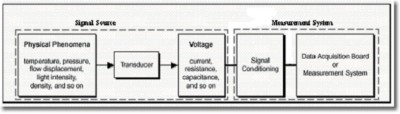Figure 1. Typical data acquisition block diagram

For a better understanding of the effect of ground on measurements, you should first study the different types of signal sources and measurement systems. It is then very important to go over different configurations to match a signal source with its best measurement system for minimal noise and error.

## Signal Sources

As it is shown on Figure 2, there are two main categories of signal source:

• Grounded or Ground-referenced
• Ungrounded or Floating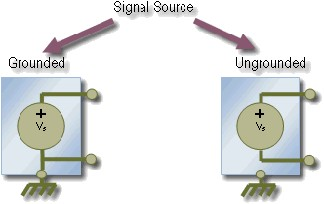Figure 2. Signal Source Types

Grounded or Ground-Referenced Signal Sources
A grounded signal source is one in which the voltage signals are referenced to a system ground, such as earth or building ground. Note that the negative terminal of the signal source shown above is referenced to ground. The most common examples of grounded signal sources are devices, such as power supplies, oscilloscopes, and signal generators that plug into the building ground through a wall outlet, as shown in figure 3.

The grounds of two independently grounded signal sources generally will not be at the same potential. The difference in ground potential between two instruments connected to the same building ground system is typically 10mV to 200mV, or even more. The difference can be higher if power distribution circuits are not properly connected.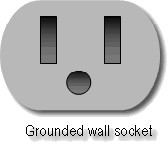Figure 3. Grounded Wall Socket

Ungrounded or Floating Signal Sources
A floating or ungrounded signal source is one in which the voltage signal is not referenced to a system ground, such as earth or building ground. Note on Figure 2 above that neither the positive nor the negative terminal is referenced to ground for the ungrounded source. Common examples of floating signal sources include the digital multimeter, batteries, thermocouples, transformers, and isolation amplifiers.

## Measurement Systems

Most measurement systems, such as a NI  Data Acquisition (DAQ) device, can be configured in one of the following modes: Differential, Reference Single Ended (RSE) or Non-Reference Single Ended (NRSE).

Differential Measurement System
In a differential measurement system neither input to the instrumentation amplifier is referenced to a system ground. In Figure 4, each channel of the measurement system has a negative and a positive lead, none of which is connected to the measurement system ground (AIGND). Measuring a signal on channel 0 in differential mode would require connecting one lead of the signal source to CH0+ and the other lead to CH0-.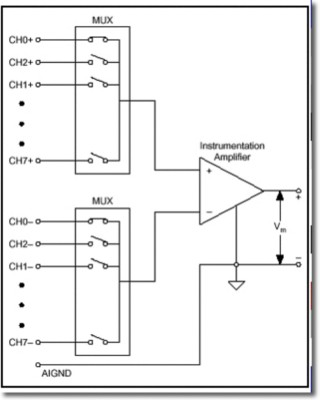Figure 4. Differential Measurement System

Measurements done in differential mode require more channels since each measurement requires two analog input channels. However, differential mode can deliver more accurate measurements, because it allows the amplifier to reject common-mode voltage and other common-mode noise present in the signal. Common-mode voltage is any voltage present at the instrumentation amplifier inputs with respect to the amplifier ground. The formula for calculating the amount of common-mode voltage with respect to the DAQ device ground is as follows:

V cm = (V + + V -) / 2

V+ and V- are the voltages at "common" to both the positive and negative terminals of the amplifier referenced to the amplifier ground. Figure 5 displays how you measure the common mode voltage by shorting V+ and V- ; therefore making the differential voltage zero. Any voltage measured at Vout is equal to the common mode voltage at the input of the amplifier.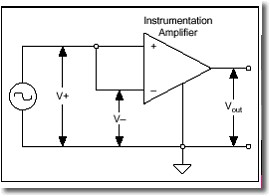Figure 5. Common Mode Voltage

An ideal differential measurement system reads only the potential difference between the positive and negative terminals of the amplifier and thus it completely rejects common-mode voltages. However, practical devices are limited in their ability to reject common-mode voltage. You can calculate the amount of common-mode voltage your system can reject with the following formula:
V cm(max) = {MVW - [(V diff(max)) x (Amplification)]}/2

• MVW is the Maximum Working Voltage listed in the device specifications
• Vdiff(max) is the maximum expected difference between the amplifier terminals
• Amplification is device gain setting

Single-Ended Measurement System
Single ended is the "default" configuration for most data acquisition devicesmodular instruments, and stand-alone devices. Figure 6a show an example of Single Ended configuration. In contrast to the Differential mode, channels in Single Ended modes require only a single analog input channel. The second lead is common and used by all channels. This configuration has the advantage that the channel count is doubled. This results from the fact that all the channels that were used in Differential mode as the negative channel inputs are now available. However, single-ended systems are very susceptible to ground loops, for more information about removing ground loops from your system please read the Measurement Configuration section below.

There are essentially two main types of Single-Ended measurement systems:

• Ground Referenced Single Ended (GRSE) or simply called Referenced Single Ended (RSE) refers to single-ended systems in which the common channel is connected to ground. Figure 6a below depicts a Reference Single Ended system in which all the channels are referenced to AIGND which represents the system ground.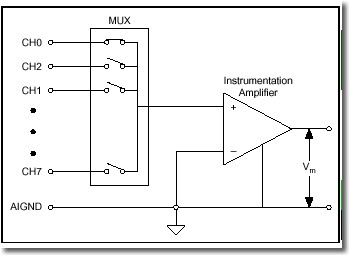Figure 6a Ground Referenced Single-Ended (GRSE) or Referenced Single Ended (RSE)

• In a Non-Referenced Single-Ended (NRSE) system, all the channels are still referenced to a common point. However, the common channel in this case is not grounded. Figure 6b below shows an example of a Non-Referenced Single Ended system where all the channels are referenced to AISENSE which is not connected to system ground.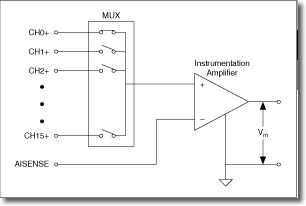Figure 6b Non-Referenced Single-Ended (NRSE)

## Signal Source - Measurement System Configurations

Measuring Grounded Signal Sources
A grounded signal source is best measured with a differential or non-referenced measurement system. Figure 7 shows the pitfall of using a ground-referenced measurement system to measure a grounded signal source. In this case, the measured voltage, Vm, is the sum of the signal voltage, Vs, and the potential difference, ΔVg, that exists between the signal source ground and the measurement system ground. This potential difference is generally not a DC level; thus, the result is a noisy measurement system often revealing power-line frequency (60 Hz) components in the readings. As mentioned earlier, there can exist up to 200 mV difference between two ground connections. This difference causes a current called ground loop current to flow in the interconnection which can greatly affect measurements causing offset errors, especially when measuring low level signals from sensors.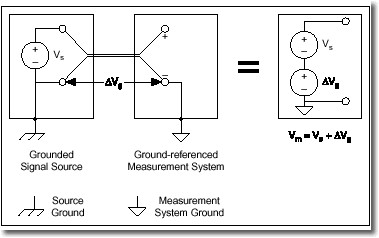Figure 7 Measurement System with a Ground Loop

A ground-referenced system is an acceptable solution if the signal voltage levels are high and the interconnection wiring between the source and the measurement device has a low impedance. In this case, the signal voltage measurement is degraded by ground loops, but the degradation may be tolerable. The polarity of a grounded signal source must be carefully observed before connecting it to a ground-referenced measurement system because the signal source can be shorted to ground, thus possibly damaging the signal source.

Measuring Floating (Non-referenced) Sources
Floating signal sources can be measured with both differential and single-ended measurement systems. In the case of the differential measurement system, however, care should be taken to ensure that the common-mode voltage level of the signal with respect to the measurement system ground remains in the common-mode input range of the measurement device. A variety of phenomena - for example, the instrumentation amplifier input bias currents - can move the voltage level of the floating source out of the valid range of the input stage of a data acquisition device. To anchor this voltage level to some reference, resistors are used. These resistors, called bias resistors, provide a DC path from the instrumentation amplifier inputs to the instrumentation amplifier ground. These resistors should be of a large enough value to allow the source to float with respect to the measurement reference (AIGND in the previously described measurement system) and not load the signal source, but small enough to keep the voltage in the range of the input stage of the device. Typically, values between 10 kΩ and 100 kΩ work well with low-impedance sources such as thermocouples and signal conditioning module outputs. These bias resistors are connected between each lead and the measurement system ground. Failure to use these resistors may result in erratic or saturated (positive full-scale or negative full-scale) readings.

If the input signal is DC-coupled, only one resistor connected from the (-) negative input to the measurement system ground is required to satisfy the bias current path requirement, but this leads to an unbalanced system if the source impedance of the signal source is relatively high. Balanced systems are desirable from a noise immunity point of view. Consequently, two resistors of equal value - one for signal high (+) input and the other for signal low (-) input to ground - should be used if the source impedance of the signal source is high. A single bias resistor is sufficient for low-impedance DC-coupled sources such as thermocouples. Balanced circuits are discussed further later in this application note. If the input signal is AC-coupled, two bias resistors are required to satisfy the bias current path requirement of the instrumentation amplifier.

If the single-ended input mode is to be used, a GRSE input system (Figure 8a) can be used for a floating signal source. No ground loop is created in this case. The NRSE input system (Figure 12b) can also be used and is preferable from a noise pickup point of view. Floating sources do require bias resistor(s) between the AISENSE input and the measurement system ground (AIGND) in the NRSE input configuration.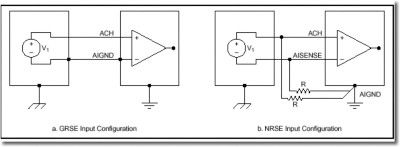Figure 8 Floating Signal Source and Single-Ended Configurations

Table 1 below shows a summary of the recommended configurations.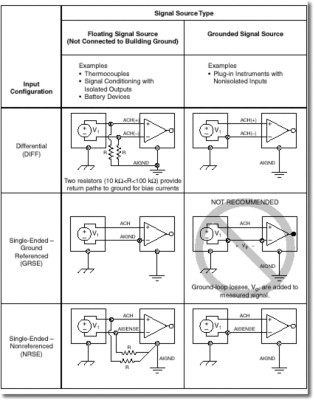## Conclusion

In general, a differential measurement system is preferable because it rejects not only ground loop-induced errors, but also the noise picked up in the environment to a certain degree. The single-ended configurations, on the other hand, provide twice as many measurement channels but are justified only if the magnitude of the induced errors is smaller than the required accuracy of the data. Single-ended input connections can be used when all input signals meet the following criteria.

• Input signals are high level (greater than 1 V as a rule of thumb)
• Signal cabling is short and travels through a noise-free environment or is properly shielded
• All input signals can share a common reference signal at the source

Differential connections should be used when any of the above criteria are violated.

## Relevant NI products

Customers interested in this topic were also interested in the following NI products: